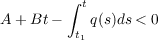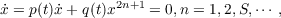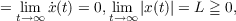#### Vol. 24, No. 2, 1968

 Download this articleFor screen For printingRecent Issues Vol. 325: 1 Vol. 324: 1  2 Vol. 323: 1  2 Vol. 322: 1  2 Vol. 321: 1  2 Vol. 320: 1  2 Vol. 319: 1  2 Vol. 318: 1  2Online Archive Volume: Issue:The Journal Subscriptions Editorial Board Officers Contacts Submission Guidelines Submission Form Policies for Authors ISSN: 1945-5844 (e-only) ISSN: 0030-8730 (print) Special Issues Author Index To Appear Other MSP Journals
A stability theorem for a third order nonlinear differential equation

### J. L. Nelson

Vol. 24 (1968), No. 2, 341–344
##### Abstract

A stability theorem and a corollary are proved for a nonlinear nonautonomous third order differential equation. A remark shows that the results do not hold for the linear case. THEOREM. Let p(t) and q(t) be continuous and q(t) 0, p(t) < 0 with p(t) 0. For any A and B supposefor large t where Q(t) = t0tq(s)ds, then any nonoscillatory solution ixj(t) of the equationhas the following properties; sgn x = sgn X, sgn ,limt→∞(t)and x(t)X(t),X(t) are monotone functions. COROLLARY. If q(t) >> 0 for large t, then limt→∞x(t) = 0.

Primary: 34.50
##### Milestones